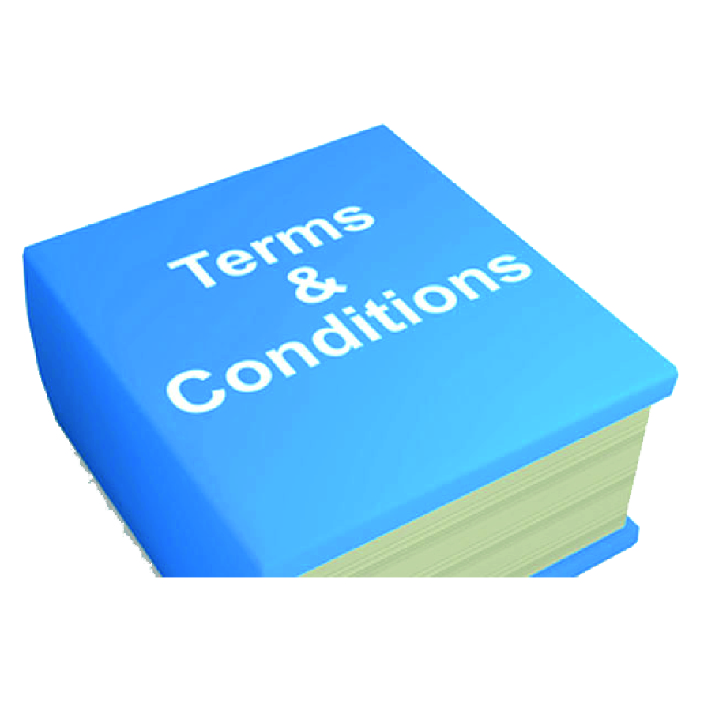Pilot request form

Invalid Input
Invalid Input
Invalid Input
Invalid Input
Invalid Input
Invalid Input
Invalid Input
Invalid Input
Invalid Input
Invalid Input
Invalid Input
Invalid Input
Invalid Input
Invalid Input
Invalid Input
Invalid Input
Invalid Input
Invalid Input
Model 1 details
Invalid Input
Invalid Input
Invalid Input
Invalid Input
Invalid Input
Invalid Input
Invalid Input
Invalid Input
Invalid Input

Model 2 details

Invalid Input
Invalid Input
Invalid Input
Invalid Input
Invalid Input
Invalid Input
Invalid Input
Invalid Input
Invalid Input

Model 3 details

Invalid Input
Invalid Input
Invalid Input
Invalid Input
Invalid Input
Invalid Input
Invalid Input
Invalid Input
Invalid Input

Model 4 details

Invalid Input
Invalid Input
Invalid Input
Invalid Input
Invalid Input
Invalid Input
Invalid Input
Invalid Input
Invalid Input

We acceptFind us onT's & C's